## 11-2 Filter Design (瞈暹郭?刻身閮?

In the previous section, we have seen some basic filters and their applications. In this section, we shall cover some basic approaches to filter design using MATLAB.

We can use the MATLAB command "butter" to design a Butterworth low-pass filter, with the following format:

[b, a] = butter(order, wn, function)
The input arguments to butter can be explained next:
• order: order of the filte. A larger order leads to a better filtering effect. However, the required computing time is also proportional to the order since the the length of the parameters a and b is equal to order+1。
• wn: normalized cutoff frequency within the range of 0 and 1. When the sample frequency is fs, the maximum allowable frequency in frequency-domain processing is fs/2. Therefore the normalized cutoff frequency wn is equal to the real cutoff frequency divided by fs/2.
• function: a string representing the filter function, which could be 'low' or 'high', representing low-pass and high-pass, respectively.

In the next example, we use the command "butter" to design a Butterworth low-pass filter.

Example 1: butter01.mfs=8000; % Sampling rate filterOrder=5; % Order of filter cutOffFreq=1000; % Cutoff frequency [b, a]=butter(filterOrder, cutOffFreq/(fs/2), 'low'); % === Plot frequency response [h, w]=freqz(b, a); plot(w/pi*fs/2, abs(h), '.-'); title('Magnitude frequency response'); grid on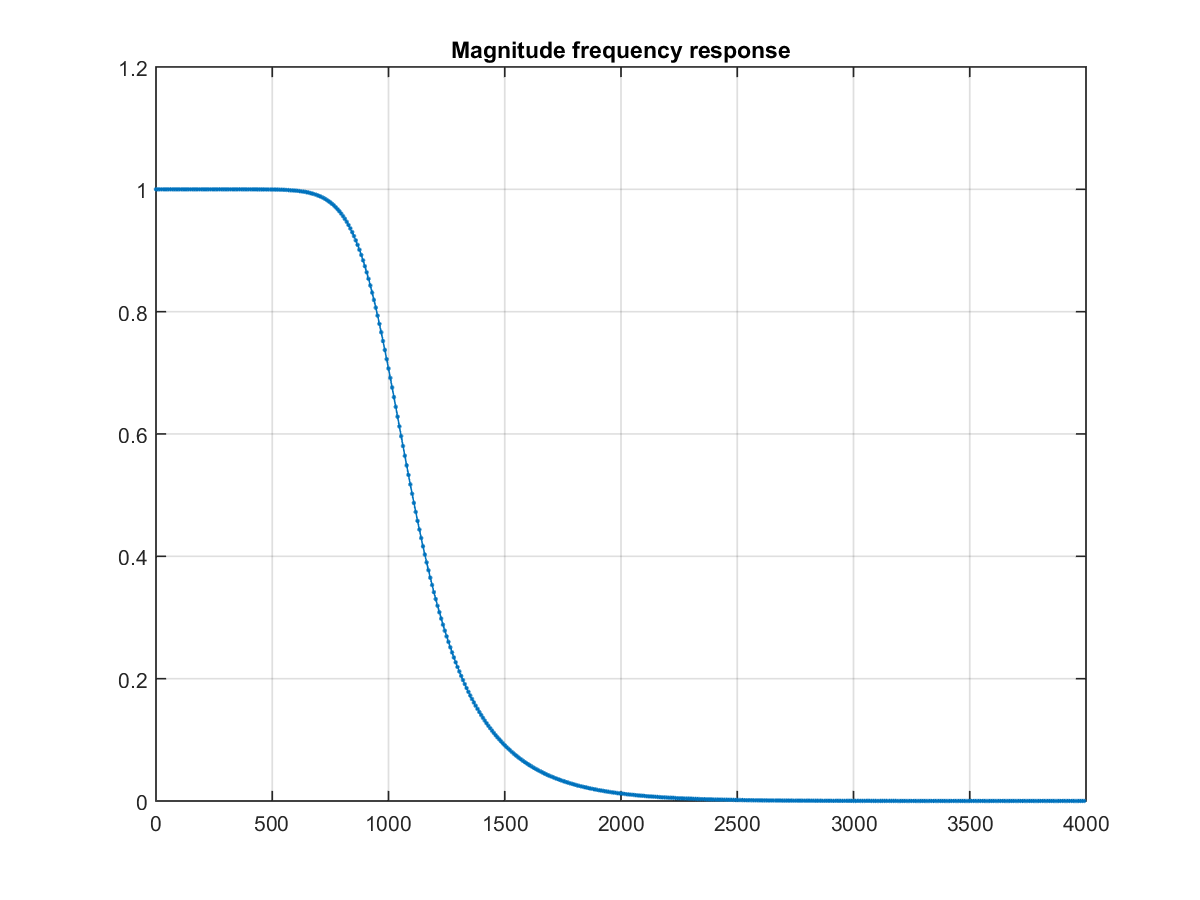In the above example, we have designed a Butterworth filter with a cutoff frequency of 1000 Hz. The plot is the magnitude frequency response of the filter.

When the order of the filter is bigger, the filtering effect is better at the cost of longer computation time. On the other hand, a smaller order leads to a shorter computation time and less desirable filtering effect. The following example demonstrates the magnitude frequency response as a function of the order of the Butterworth filter.

Example 2: butter02.mfs=8000; % Sampling rate cutOffFreq=1000; % Cutoff frequency allH=[]; for filterOrder=1:8; [b, a]=butter(filterOrder, cutOffFreq/(fs/2), 'low'); % === Plot frequency response [h, w]=freqz(b, a); allH=[allH, h]; end plot(w/pi*fs/2, abs(allH)); title('Frequency response of a low-pass utterworth filter'); legend('order=1', 'order=2', 'order=3', 'order=4', 'order=5', 'order=6', 'order=7', 'order=8');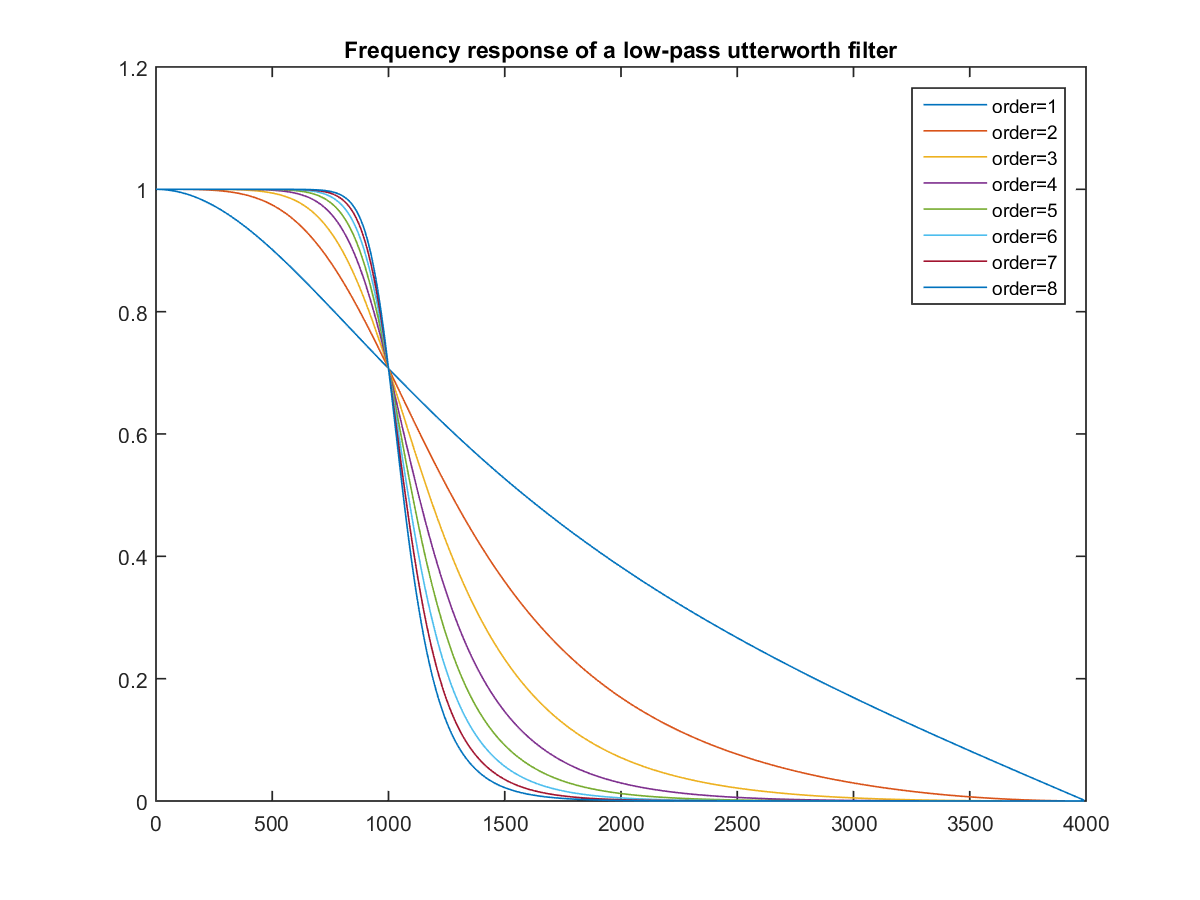As it is obvious in the above example, when the order is increased from 1 to 8, the magnitude frequency response is becoming sharper at the cutoff frequency of 1000 Hz.

We can apply the above filter to a clip of a pop song to see if the high-frequency components can be removed. See the next example.

Example 3: butter03.mcutOffFreq=1000; % Cutoff frequency filterOrder=5; % Order of filter au=myAudioRead('wubai_solicitude.wav'); [b, a]=butter(filterOrder, cutOffFreq/(au.fs/2), 'low'); au.signal=au.signal(60*au.fs:90*au.fs); % 30-second signal y=filter(b, a, au.signal); % ====== Plot the result time=(1:length(au.signal))/au.fs; subplot(2,1,1); plot(time, au.signal); subplot(2,1,2); plot(time, y); % ====== Save output files myAudioWrite(au, 'wubai_solicitude_orig.wav'); au.signal=y; myAudioWrite(au, sprintf('wubai_solicitude_%d.wav', cutOffFreq)); [Warning: Data clipped when writing file.] [> In <a href="matlab:matlab.internal.language.introspective.errorDocCallback('audiowrite>clipInputData', 'E:\MATLAB\R2015a\toolbox\matlab\audiovideo\audiowrite.m', 396)" style="font-weight:bold">audiowrite>clipInputData</a> (<a href="matlab: opentoline('E:\MATLAB\R2015a\toolbox\matlab\audiovideo\audiowrite.m',396,0)">line 396</a>) In <a href="matlab:matlab.internal.language.introspective.errorDocCallback('audiowrite', 'E:\MATLAB\R2015a\toolbox\matlab\audiovideo\audiowrite.m', 176)" style="font-weight:bold">audiowrite</a> (<a href="matlab: opentoline('E:\MATLAB\R2015a\toolbox\matlab\audiovideo\audiowrite.m',176,0)">line 176</a>) In <a href="matlab:matlab.internal.language.introspective.errorDocCallback('myAudioWrite', 'd:\users\jang\matlab\toolbox\sap\myAudioWrite.m', 29)" style="font-weight:bold">myAudioWrite</a> (<a href="matlab: opentoline('d:\users\jang\matlab\toolbox\sap\myAudioWrite.m',29,0)">line 29</a>) In <a href="matlab:matlab.internal.language.introspective.errorDocCallback('butter03', 'D:\users\jang\books\audioSignalProcessing\example\butter03.m', 16)" style="font-weight:bold">butter03</a> (<a href="matlab: opentoline('D:\users\jang\books\audioSignalProcessing\example\butter03.m',16,0)">line 16</a>) In <a href="matlab:matlab.internal.language.introspective.errorDocCallback('goWriteOutputFile>dummyFunction', 'D:\users\jang\books\goWriteOutputFile.m', 85)" style="font-weight:bold">goWriteOutputFile>dummyFunction</a> (<a href="matlab: opentoline('D:\users\jang\books\goWriteOutputFile.m',85,0)">line 85</a>) In <a href="matlab:matlab.internal.language.introspective.errorDocCallback('goWriteOutputFile', 'D:\users\jang\books\goWriteOutputFile.m', 55)" style="font-weight:bold">goWriteOutputFile</a> (<a href="matlab: opentoline('D:\users\jang\books\goWriteOutputFile.m',55,0)">line 55</a>)]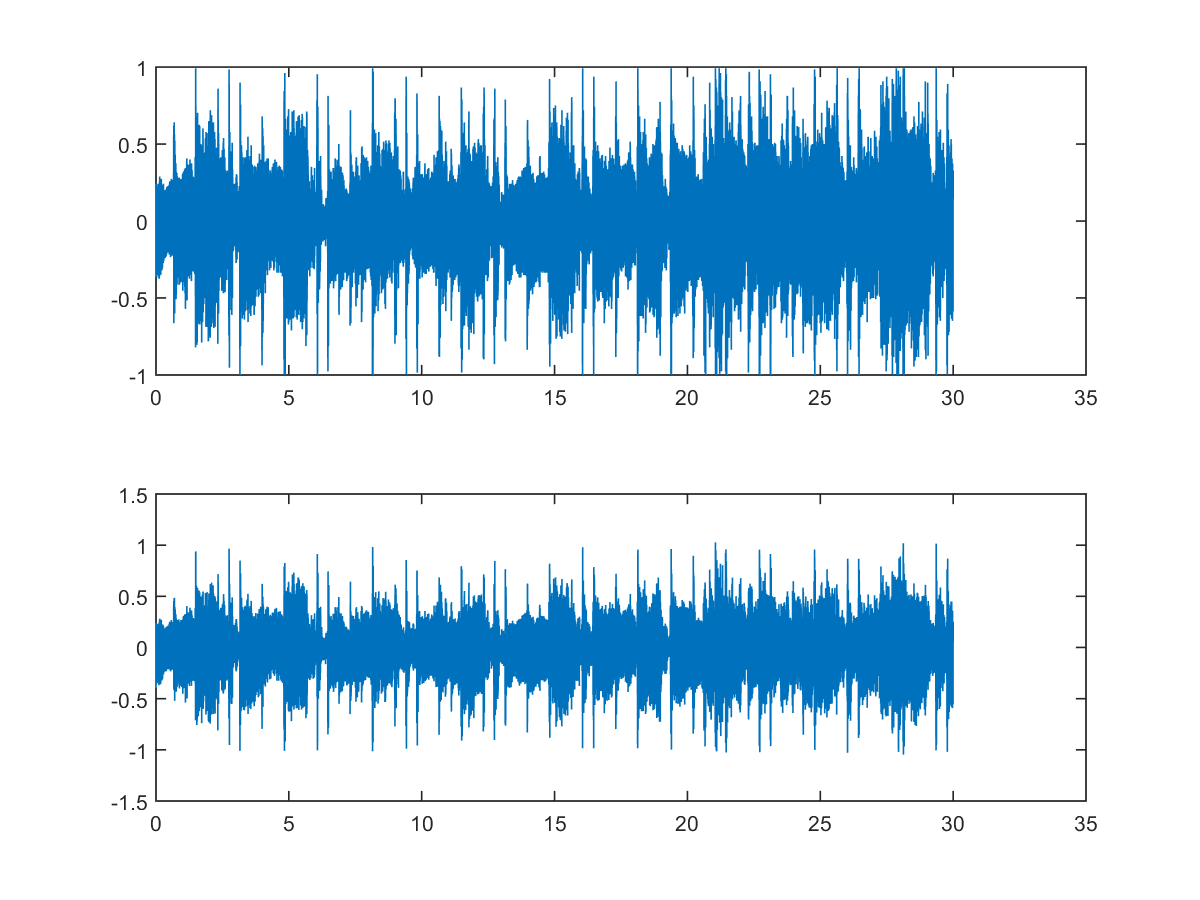We can hear the original and the output clips:
The playback of the output signal demonstrates that the high-frequency components are eliminated from the original signal.

If we set up the cutoff frequency to 100 Hz, then the output signal is almost inaudible unless we have a speaker with a subwoofer. See the next example.

Example 4: butter04.mcutOffFreq=100; % Cutoff freq (截止頻率) filterOrder=5; % Order of filter (濾波器的階數) au=myAudioRead('wubai_solicitude.wav'); [b, a]=butter(filterOrder, cutOffFreq/(au.fs/2), 'low'); au.signal=au.signal(60*au.fs:90*au.fs); % 30 seconds of singing (30 秒歌聲) y=filter(b, a, au.signal); % ====== Plotting (畫圖) time=(1:length(au.signal))/au.fs; subplot(2,1,1); plot(time, au.signal); subplot(2,1,2); plot(time, y); % ====== Save wav files (存檔) myAudioWrite(au, 'wubai_solicitude_orig.wav'); au.signal=y; myAudioWrite(au, sprintf('wubai_solicitude_%d.wav', cutOffFreq)); [Warning: Data clipped when writing file.] [> In <a href="matlab:matlab.internal.language.introspective.errorDocCallback('audiowrite>clipInputData', 'E:\MATLAB\R2015a\toolbox\matlab\audiovideo\audiowrite.m', 396)" style="font-weight:bold">audiowrite>clipInputData</a> (<a href="matlab: opentoline('E:\MATLAB\R2015a\toolbox\matlab\audiovideo\audiowrite.m',396,0)">line 396</a>) In <a href="matlab:matlab.internal.language.introspective.errorDocCallback('audiowrite', 'E:\MATLAB\R2015a\toolbox\matlab\audiovideo\audiowrite.m', 176)" style="font-weight:bold">audiowrite</a> (<a href="matlab: opentoline('E:\MATLAB\R2015a\toolbox\matlab\audiovideo\audiowrite.m',176,0)">line 176</a>) In <a href="matlab:matlab.internal.language.introspective.errorDocCallback('myAudioWrite', 'd:\users\jang\matlab\toolbox\sap\myAudioWrite.m', 29)" style="font-weight:bold">myAudioWrite</a> (<a href="matlab: opentoline('d:\users\jang\matlab\toolbox\sap\myAudioWrite.m',29,0)">line 29</a>) In <a href="matlab:matlab.internal.language.introspective.errorDocCallback('butter04', 'D:\users\jang\books\audioSignalProcessing\example\butter04.m', 16)" style="font-weight:bold">butter04</a> (<a href="matlab: opentoline('D:\users\jang\books\audioSignalProcessing\example\butter04.m',16,0)">line 16</a>) In <a href="matlab:matlab.internal.language.introspective.errorDocCallback('goWriteOutputFile>dummyFunction', 'D:\users\jang\books\goWriteOutputFile.m', 85)" style="font-weight:bold">goWriteOutputFile>dummyFunction</a> (<a href="matlab: opentoline('D:\users\jang\books\goWriteOutputFile.m',85,0)">line 85</a>) In <a href="matlab:matlab.internal.language.introspective.errorDocCallback('goWriteOutputFile', 'D:\users\jang\books\goWriteOutputFile.m', 55)" style="font-weight:bold">goWriteOutputFile</a> (<a href="matlab: opentoline('D:\users\jang\books\goWriteOutputFile.m',55,0)">line 55</a>)]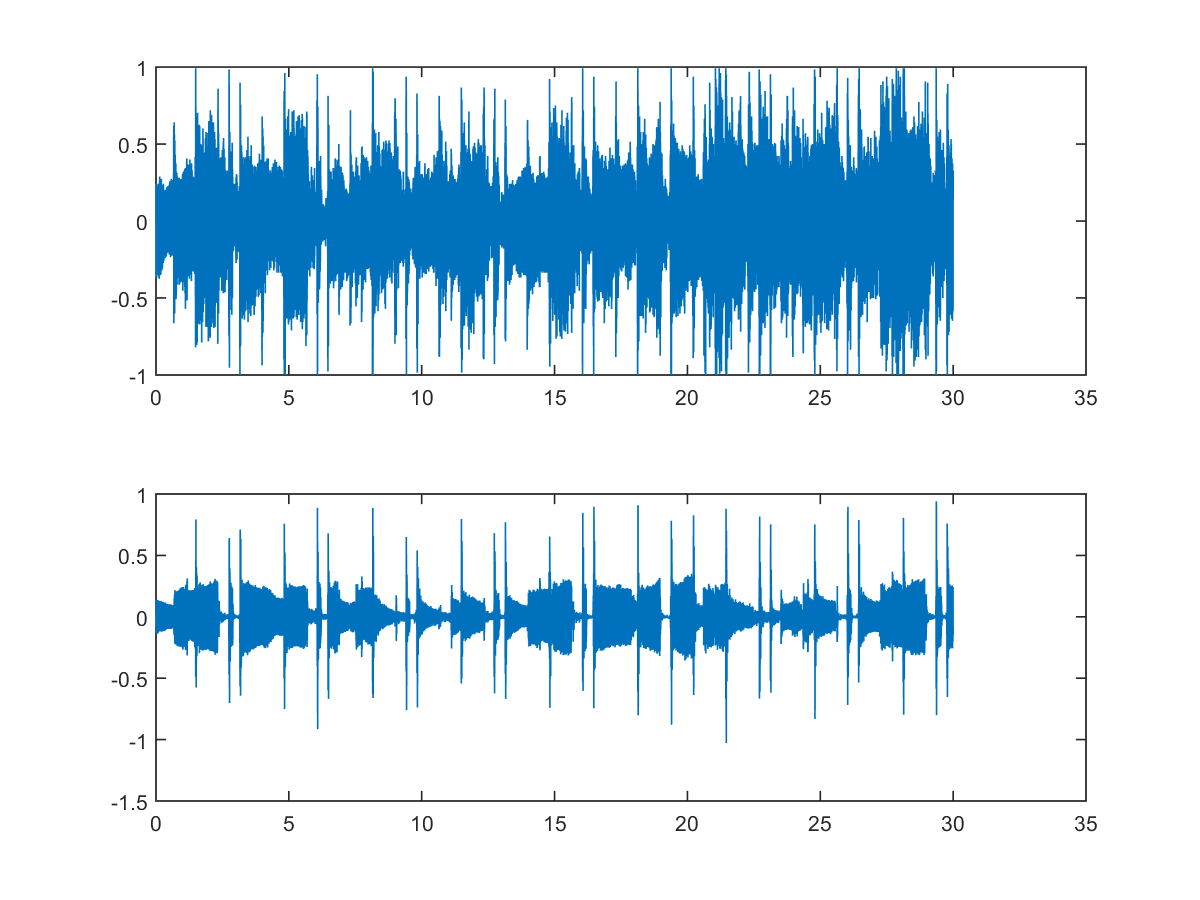Obviously, after the low-pass filter at a cutoff frequency of 100 Hz, most of the sounds are removed except for those from the bass drum.

Hint
In fact, the periodical sounds of the bass drum can help us tracking the beat of the music. This problem of beat tracking is an active research topic in the literature of music information retrieval.

If you cannot identify the sounds of the bass drum, you can hear the playback of the following clips one by one. (If you use CoolEdit for playback, the progress bar can help you identify where the sounds of the bass drum are.)

In fact, by supplying appropriates input parameters, we can use the command "butter" to design four types of filters, including low-pass, high-pass, band-pass, band-stop filters. The following example plots typical frequency responses of these filters.

Example 5: butter05.mfs=8000; % Sampling rate filterOrder=5; % Order of filter % ====== low-pass filter cutOffFreq=1000; [b, a]=butter(filterOrder, cutOffFreq/(fs/2), 'low'); [h, w]=freqz(b, a); subplot(2,2,1); plot(w/pi*fs/2, abs(h), '.-'); xlabel('Freq (Hz)'); title('Freq. response of a low-pass filter'); grid on % ====== high-pass filter cutOffFreq=2000; [b, a]=butter(filterOrder, cutOffFreq/(fs/2), 'high'); [h, w]=freqz(b, a); subplot(2,2,2); plot(w/pi*fs/2, abs(h), '.-'); xlabel('Freq (Hz)'); title('Freq. response of a high-pass filter'); grid on % ====== band-pass filter passBand=[1000, 2000]; [b, a]=butter(filterOrder, passBand/(fs/2)); [h, w]=freqz(b, a); subplot(2,2,3); plot(w/pi*fs/2, abs(h), '.-'); xlabel('Freq (Hz)'); title('Freq. response of a band-pass filter'); grid on % ====== band-stop filter stopBand=[1000, 2000]; [b, a]=butter(filterOrder, stopBand/(fs/2), 'stop'); [h, w]=freqz(b, a); subplot(2,2,4); plot(w/pi*fs/2, abs(h), '.-'); xlabel('Freq (Hz)'); title('Freq. response of a band-stop filter'); grid on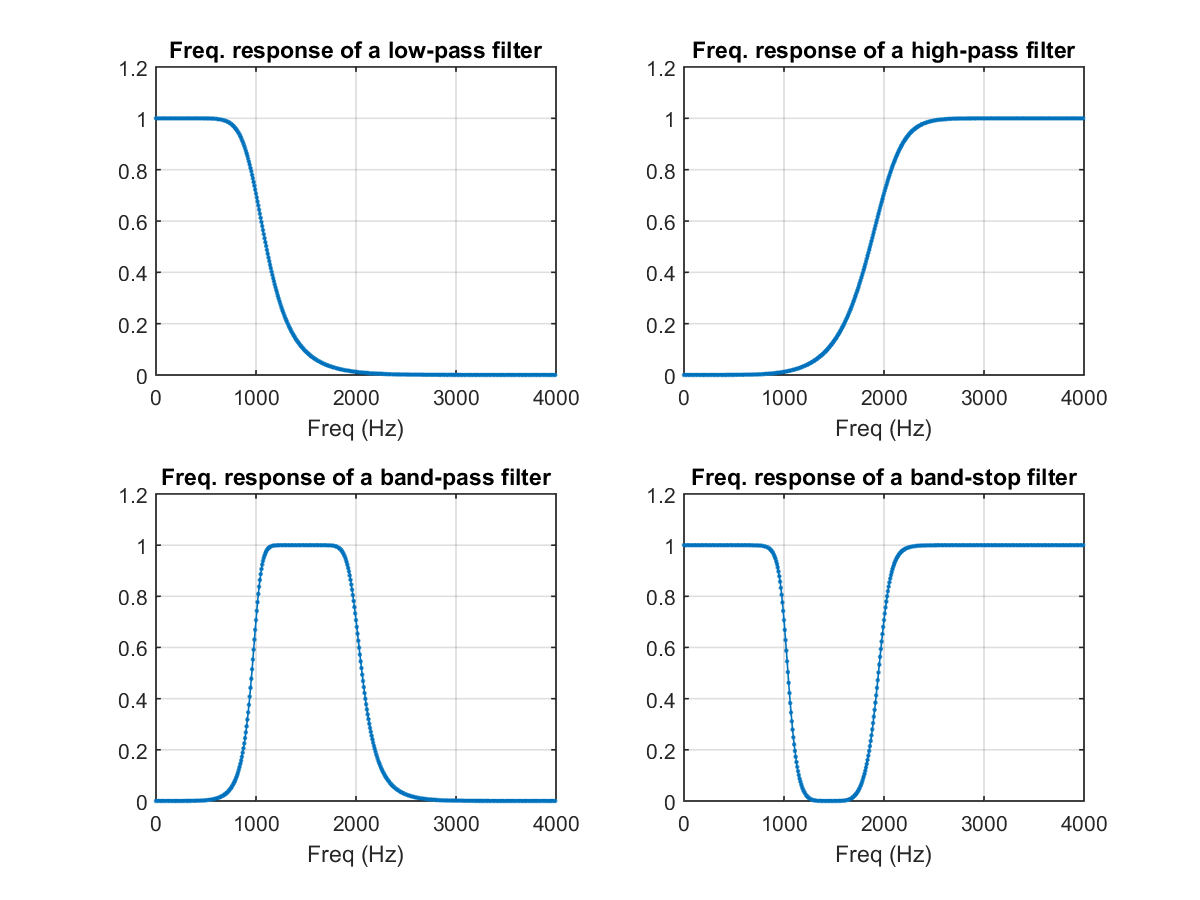Audio Signal Processing and Recognition (音訊處理與辨識)